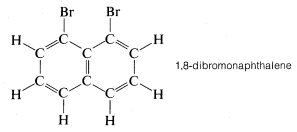# 2.2: The Sizes and Shapes of Organic Molecules

$$\newcommand{\vecs}{\overset { \rightharpoonup} {\mathbf{#1}} }$$ $$\newcommand{\vecd}{\overset{-\!-\!\rightharpoonup}{\vphantom{a}\smash {#1}}}$$$$\newcommand{\id}{\mathrm{id}}$$ $$\newcommand{\Span}{\mathrm{span}}$$ $$\newcommand{\kernel}{\mathrm{null}\,}$$ $$\newcommand{\range}{\mathrm{range}\,}$$ $$\newcommand{\RealPart}{\mathrm{Re}}$$ $$\newcommand{\ImaginaryPart}{\mathrm{Im}}$$ $$\newcommand{\Argument}{\mathrm{Arg}}$$ $$\newcommand{\norm}{\| #1 \|}$$ $$\newcommand{\inner}{\langle #1, #2 \rangle}$$ $$\newcommand{\Span}{\mathrm{span}}$$ $$\newcommand{\id}{\mathrm{id}}$$ $$\newcommand{\Span}{\mathrm{span}}$$ $$\newcommand{\kernel}{\mathrm{null}\,}$$ $$\newcommand{\range}{\mathrm{range}\,}$$ $$\newcommand{\RealPart}{\mathrm{Re}}$$ $$\newcommand{\ImaginaryPart}{\mathrm{Im}}$$ $$\newcommand{\Argument}{\mathrm{Arg}}$$ $$\newcommand{\norm}{\| #1 \|}$$ $$\newcommand{\inner}{\langle #1, #2 \rangle}$$ $$\newcommand{\Span}{\mathrm{span}}$$$$\newcommand{\AA}{\unicode[.8,0]{x212B}}$$

The size and shape of molecules are as much a part of molecular structure as is the order in which the component atoms are bonded. Contrary to the impression you may get from structural formulas, complex molecules are not flat and formless, but have well-defined spatial arrangements that are determined by the lengths and directional character of their chemical bonds. It is not easy to visualize the possible arrangements of the bonds in space and it is very helpful to have some kind of mechanical model that reflects the molecular geometry, including at least an approximation to the relative lengths of the bonds. "Ball-and-stick" models such as the ones used by Paterno (Section 1-1D) fill this purpose admirably.

## Bond Angles and Ball-and-Stick Models

It is well established that the normal carbon atom forms its four single bonds in compounds of the type $$CX_4$$ so that the four attached atoms lie at the corners of a regular tetrahedron. The bond angles $$X-C-X$$ are $$109.5^\text{o}$$ and this value is the "normal" valence angle of carbon. For many purposes, ball-and-stick models of organic compounds give useful information about the spatial relationships of the atoms, and for $$CX_4$$ the angles between sticks are set at $$109.5^\text{o}$$ (Figure 2-1). Organic molecules strongly resist deformation forces that alter their valence angles from normal values. Therefore ball-and-stick models correspond better to the behavior of actual molecules if the connectors representing single bonds are made to be rather stiff.Figure 2-1: Ball-and-stick model of $$CX_4$$Figure 2-2: Ball-and-stick models of some simple organic molecules

Whereas methane, $$CH_4$$, is tetrahedral, ethene, $$C_2H_4$$, is not. According to the best available physical measurements, all six atoms of ethene lie in a single plane and the $$H-C-H$$ bond angles are $$117.3^\text{o}$$. Methanal (formaldehyde) also is a planar molecule with an $$H-C-H$$ bond angle of $$118^\text{o}$$.Figure 2-2), but the $$H-C-H$$ angles are inaccurate because they are $$109.5^\text{o}$$ rather than the observed $$117^\text{o}$$ to $$118^\text{o}$$.

Ethyne, $$C_2H_2$$, has been established experimentally to be a linear molecule; that is, the $$H-C-C$$ bond angle is $$180^\text{o}$$:

Structural units that have $$C-C-C$$ valence angles substantially less than the tetrahedral value include double and triple bonds, and small rings such as cyclopropane. Several bent bonds are required to construct models of compounds containing these units. Interestingly, such compounds are much less stable and more reactive than otherwise similar molecules for which models can be constructed with straight sticks at tetrahedral angles.

## Bond Lengths and Space-Filling Models

The length of a chemical bond is the average distance between the nuclei of two bonded atoms, regardless of where the bonding electrons happen to be. The customary unit of length is the angstrom$$^1$$ ($$\text{A} = 10^{-10} \: \text{m}$$), and measurements often can be made with an accuracy of $$0.001 \: \text{A}$$ by using the techniques of molecular spectroscopy, x-ray diffraction (for crystalline solids), and electron diffraction (for volatile compounds). Bond lengths vary considerably with structure and depend on the identity of both atoms, the type of bonding (single, double, or triple), and the nature of other atoms or groups bonded to the two atoms in question. These effects are apparent in the data of Table 2-1, which lists the bond lengths in several simple organic compounds. Multiple bonds, double or triple, clearly are shorter than single bonds, and it can be stated as a general observation that the more bonding electrons in a given bond, the shorter (and stronger) the bond. The lengths of single $$C-C$$ bonds also vary significantly depending on what other atoms or groups are attached to the carbons. Thus Table 2-1 shows that single $$C-C$$ bonds become progressively shorter as the number of multiple bonds or electronegative atoms attached to the carbons increases.

Although molecular models cannot represent the subtle variations in bond lengths and bond angles that actual molecules exhibit, most kinds of commercially available molecular models do attempt to reproduce relative bond lengths with some degree of reality. In the ball-and-stick type, the sticks usually come in various lengths to simulate different kinds of bonds; $$C-H$$ bonds typically are scaled to represent $$1.1 \: \text{A}$$, $$C-C$$ bonds to be $$1.54 \: \text{A}$$, and $$C=C$$ and $$C \equiv C$$ to be correspondingly shorter. In some model sets the bonds can be cut to any desired length.

While the ball-and-stick models of molecules are very useful for visualizing the relative positions of the atoms in space, they are unsatisfactory whenever we also want to show how large the atoms are. Actually, atomic radii are so large relative to the lengths of chemical bonds that when a model of a molecule such as methyl chloride is constructed with atomic radii and bond lengths, both to scale, the bonds connecting the atoms are not clearly evident. None-Figure 2-3 shows how the CPK models can indicate intense molecular crowding, as between the bromines in 1,8-dibromonaphthalene, a close relative of 1,2-dibromobenzene mentioned in Section 1-1G:As we shall see, such crowding has many chemical consequences.

Ideally, a model should reflect not only the size and shape of the molecule it represents but also the flexibility of the molecule. By this we mean that

$$^1$$The angstrom unit likely will be replaced eventually by the nanometer ($$1 \: \text{nm} = 10^{-9} \: \text{m} = 10 \: \text{A}$$).